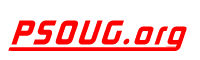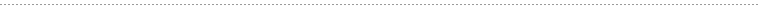Quick Search:# Term: AVG

Definition:
The Oracle AVG function returns the average of a column of numbers. An average is obtained by adding all of the values in a set and then dividing by the number of values in the set.

Example Syntax:

```SELECT AVG( value)
FROM table(s)
WHERE predicates;```

The value parameter is typically a single column in a table, but can also be an expression or formula which is evaluated. For example, multiple columns in one or more tables could be summed and then averaged.

Example Usage:

`SELECT AVG(price) FROM product_table;`

In the example above, the AVG() function returns the average cost of all of the values in the price column.

This is an example of using a formula with the AVG() function to compute the average mileage expense:

```SELECT AVG(mileage *  0.30) as "Average Mileage Expense"
FROM vehicle_list;```